# Customize plots

### Plot type

The option `type` allows to control how the data should be represented.

#### Scatter plots

In scatter plots `type` can be any combination of: points `'p'`, lines `'l'`, smooth `'s'`, text `'t'`.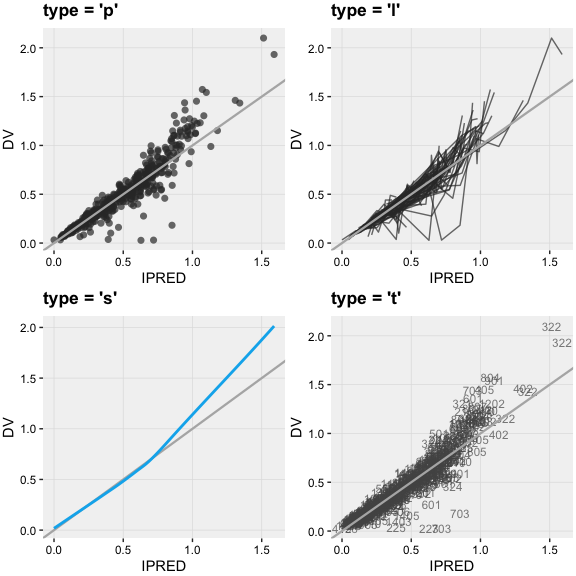#### Distribution plots

In distribution plots `type` can be any combination of: histogram `'h'`, density `'d'`, or rug `'r'`.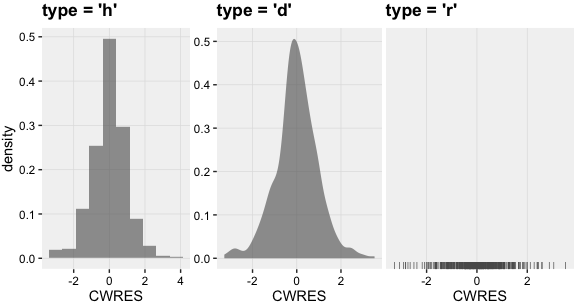#### VPCs

In visual predictive checks plots `type` can be any combination of: areas `'a'`, lines `'l'`, points `'p'`, text `'t'` or rug `'r'`.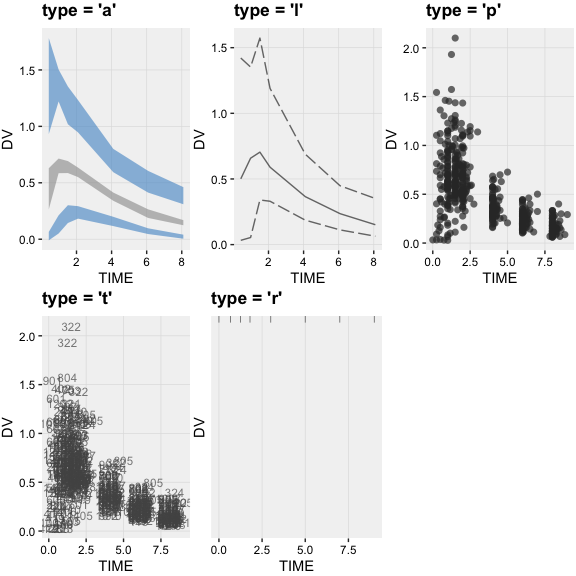### Visual guide

The option `guide` can enable (`TRUE`) or disable (`FALSE`) the visual guide on the graphs such as the line of identity on the `dv_vs_ipred()` type plot or horizontal lines on residual plots (e.g. `res_vs_idv()`).

### Labels

All xpose plots have by default an informative title, subtitle and caption. For example all plots using individual model predictions (IPRED) will display the epsilons’ shrinkage. These titles can easily be edited as templates using @keywords which will be replaced by their actual value stored in the summary level of the `xpdb` object when rendering the plots. Keywords are defined by a word preceded by a `@` e.g. `'@ofv'`. A list of all available keyword can be accessed via `help('template_titles')`. The title, subtitle or caption can be disabled by setting them to `NULL`. Suffix can be automatically added to title, subtitle and caption of all plots. The suffixes can be defined in the `xp_theme`.

#### From the plot functions

``````# Using template titles
dv_vs_ipred(xpdb,
title    = '@y vs. @x (@run, obj: @ofv)',
subtitle = 'Based on: @nind subjects and @nobs records',
caption  = 'Dir: @dir')``````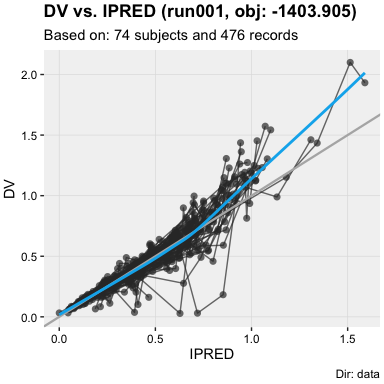``````# Disabling all titles
dv_vs_ipred(xpdb, title = NULL, subtitle = NULL, caption = NULL)``````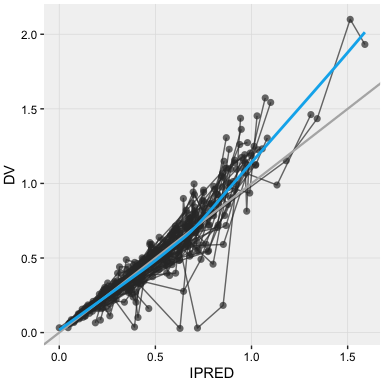``````# Edit title suffix from the xp_theme for a specific plot
dv_vs_ipred(xpdb, title = 'A title', xp_theme = list(title_suffix = ' | a suffix for @run'))``````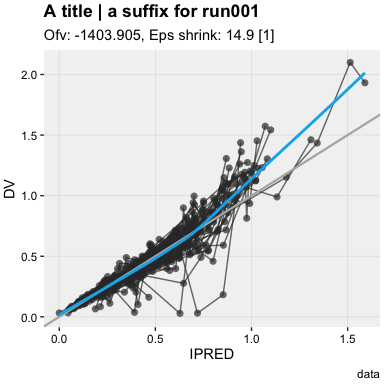#### Using the `labs` function

Labels can also modified later on by using the `ggplot2::labs()` function in combination with the ggplot2 `+` operator.

``````dv_vs_ipred(xpdb) +
labs(title    = '@descr',
subtitle = NULL,
caption  = 'Based on @nobs observations',
x        = 'Individual model predictions (@x)',
y        = 'Observations (@y) for @nind subjects')``````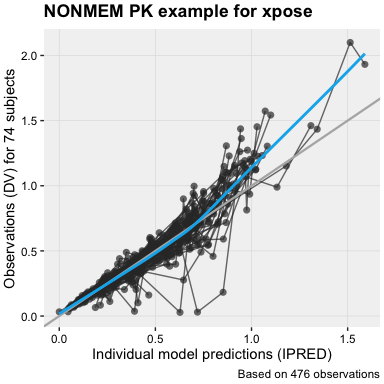### Modify aesthetics

By default the aesthetics are read from the `xp_theme` level in the xpdb object but these can be modified in any plot function. xpose makes use of the `ggplot2` functions mapping for any layer (e.g. points, lines, etc.) however to direct the mapping to a specific layer, a prefix appealing to the targeted layer should be used. The format is defined as `layer_aesthetic = value`. Hence to change the color of points in ggplot2 the argument `color = 'green'` could be used in `geom_point()`, while in xpose the same could be achieved with `point_color = 'green'`.

In basic goodness-of-fit plots, the layers have been named as: `point_xxx`, `line_xxx`, `smooth_xxx`, `guide_xxx`, `xscale_xxx`, `yscale_xxx` where `xxx` can be any option available in the `ggplot2` layers: `geom_point`, `geom_line`, `geom_smooth`, `geom_abline`, `scale_x_continuous`, etc.

``````dv_vs_ipred(xpdb,
# Change points aesthetics
point_color = 'blue', point_alpha = 0.5,
point_stroke = 0, point_size = 1.5,
# Change lines aesthetics
line_alpha = 0.5, line_linewidth = 0.5,
line_color = 'orange', line_linetype = 'solid',
# Change smooth aesthetics
smooth_method = 'lm')``````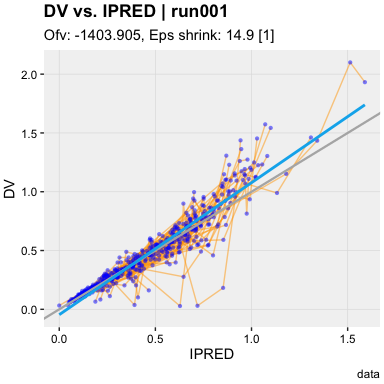Aesthetics can also be defined mapped to a variable using the ggplot2 `aes()` function.

``dv_vs_ipred(xpdb, type = 'p', aes(point_color = SEX))``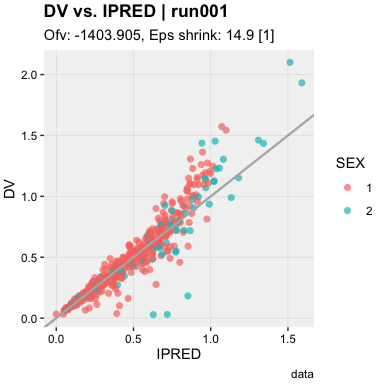### Grouping variable

The argument group defines the grouping variable to be used in the dataset for the lines only, by default group is defined to the column `'ID'`. To apply a grouping variable on any other layer, a manual mapping can be made using the argument `xxx_group = variable`

### Panels

Panels (or faceting) can be created by using the `facets` argument as follows:

• If `facets` is a string e.g `facets = "SEX"`, `ggforce::facet_wrap_paginate` will be used
• If `facets` is a formula e.g `facets = SEX~MED1`, `ggforce::facet_grid_paginate` will be used
``````# Example with a string
dv_vs_ipred(xpdb, facets = c('SEX', 'MED1'), ncol = 2, nrow = 1, page = 1)``````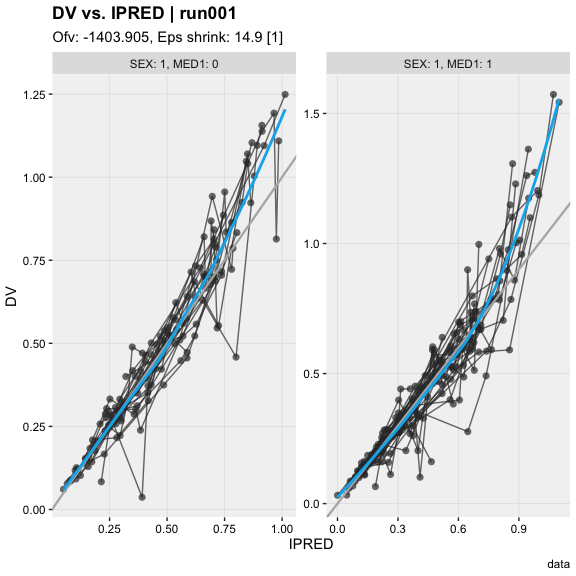``````# Example with a formula
dv_vs_ipred(xpdb, facets = SEX~MED1, margins = TRUE)``````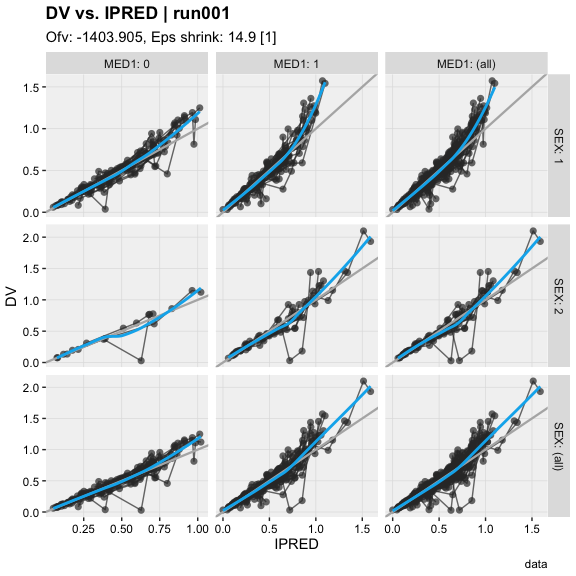All xpose plot functions accept arguments for `facet_wrap_pagninate` and `facet_grid_paginate` (e.g. `ncol = 2`, `labeller = 'label_both'`, etc.). With the default xpose theme scales are set to `'free'` from one panel to another (`scales = 'free'`), this behavior can be changed with `scales = 'fixed'`, `'free_y'` or `'free_x'`.

For more information on the available faceting options read the help of `facet_wrap_paginate` and `facet_grid_paginate` from the package ggforce.

`xpose` offers the opportunity to add any additional layers from `ggplot2`. Hence, a `ggplot2::geom_rug()` layer could be added to the `dv_vs_ipred()` plot along with some annotations (`ggplot2::annotate()`). Note: the additional layers do not inherit from the xpose aesthetic mapping (i.e. colors or other options need to be defined in each layer as shown below).

Layers can also be used to modify the aesthetics scales for example `ggplot2::scale_color_manual()`, or remove a legend `ggplot2::scale_fill_identity()`.

``````dv_vs_ipred(xpdb) +
geom_rug(alpha = 0.2, color = 'grey50',
sides = 'lb', linewidth = 0.4) +
annotate(geom = 'text',
fontface = 'bold',
color = 'darkred',
size  = 3,
label = 'Toxic concentrations', x = 1.35, y = 1.75) +
annotate(geom = 'rect',
alpha = 0.2, fill = 'red',
xmin = 1, xmax = Inf,
ymin = 1, ymax = Inf)``````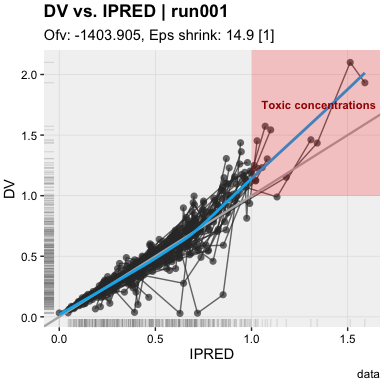### Scales options

The argument `log` allows to log-transform the axes. Accepted values are `x`, `y` or `xy`.

``dv_vs_ipred(xpdb, log = 'xy', subtitle = 'Plot on log scale')``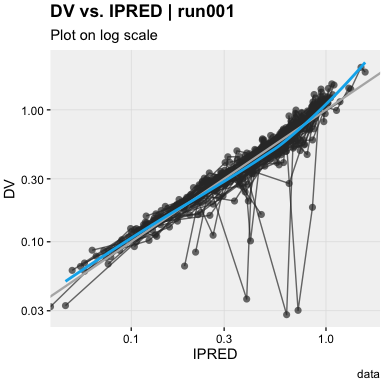Additional arguments can be provided to the scales via the mapping by using the naming convention `xscale_xxx` or `yscale_xxx` where `xxx` is the name of a `ggplot2` scale argument such as `name`, `breaks`, `labels`, `expand`.

``````dv_vs_ipred(xpdb,
xscale_breaks = c(0.3, 0.8, 1.3),
xscale_labels = c('Low', 'Med', 'High'),
xscale_expand = c(0.2, 0),
xscale_name = 'Individual model predictions')``````